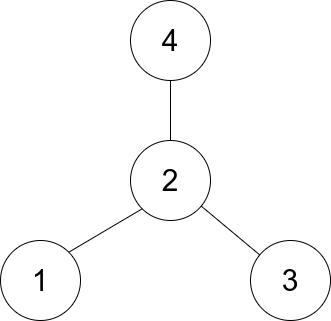# 1791. Find Center of Star Graph

Easy
There is an undirected star graph consisting of `n` nodes labeled from `1` to `n`. A star graph is a graph where there is one center node and exactly `n - 1` edges that connect the center node with every other node.
You are given a 2D integer array `edges` where each `edges[i] = [ui, vi]` indicates that there is an edge between the nodes `ui` and `vi`. Return the center of the given star graph.
Example 1:Input: edges = [[1,2],[2,3],[4,2]]
Output: 2
Explanation: As shown in the figure above, node 2 is connected to every other node, so 2 is the center.
Example 2:
Input: edges = [[1,2],[5,1],[1,3],[1,4]]
Output: 1
Constraints:
• `3 <= n <= 10^5`
• `edges.length == n - 1`
• `edges[i].length == 2`
• `1 <= ui, vi <= n`
• `ui != vi`
• The given `edges` represent a valid star graph.

### 解題

func findCenter(edges [][]int) int {
n := len(edges)
indegree := make([]int, n + 1)
for _, edge := range edges {
indegree[edge - 1]++
indegree[edge - 1]++
if indegree[edge - 1] == n {
return edge
}
if indegree[edge - 1] == n {
return edge
}
}
return 0
}